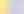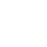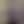25 August 2023 - 5 minutes

# Exploring Machine Learning Algorithms: From Regression to Clustering

There’s a right algorithm out there–you just need to find it.Juliette Erath - Tech Writer

Data AnalyticsYou know machine learning involves computers solving problems that would take humans a lot of time and effort, right? You’re halfway there then: learning about machine learning algorithms are the next step to understanding how to use machine learning to your advantage. In this article, we’ll cover the general importance of machine learning algorithms and the most popular ones that can help you make the most of your machine learning tools.

## What are Machine Learning Algorithms?

Machine learning algorithms are simple: they’re mapping methods used to learn about and identify underlying patterns in sets of data. As Arthur Samuel put it, machine learning is “a computer’s ability to learn without being explicitly programmed” and as computers continue to process and sort more and more data sets, they learn more and more about the best ways to handle said data.

### Why are machine learning algorithms important?

We know that machine learning algorithms use past data to predict future outcomes, which can lead to:

• Higher optimization rates

• Effective fraud detection

• Better decision making

• Improved disease/treatment prediction

• Proper sales predictions

There are four types of machine learning methods, within which the main kinds of models fall: supervised, semi-supervised, unsupervised, and reinforcement learning.

### Supervised learning

Supervised learning sounds just like it is: machines that are fed a known data set by an expert, with included desired inputs and outputs. From there, the machine has to decide how to arrive at those inputs and outputs through identifying patterns in the data, making observations to arrive at predictions.

In this case, the expert corrects the machine if needed and continues to work with it until it can guarantee high rates of accuracy. Experts using the supervised learning method can choose from three different algorithms:

• Classification: when using the classification algorithm, the program uses observed values to sort data into given categories. To do so, it uses observational data and improves the accuracy of its sorting as it gets more and more practice.

• Regression: when using the regression algorithm, the program is asked to estimate and understand variable relationships, using one dependent variable and other changing variables to predict what will happen.

• Forecasting: when using the forecasting algorithm, the program evaluates past and present data to predict future outcomes; it also analyzes trends to forecast the future.

### Semi-supervised learning

There’s one key difference between supervised and semi-supervised learning: in semi-supervised learning, programs are fed both labeled and unlabeled data so that the program must take the labeled data and draw conclusions from the unlabeled data, drawing conclusions on patterns, trends, and future predictions.

### Unsupervised learning

Just as the title suggests, unsupervised learning means the machine learning program works on its own, without labeled data or an expert, to find patterns and trends in the data. It’s given large data sets and organizes the information as it sees fit; as it receives more and more data, it’s able to become better at sorting the data effectively and properly.

Two examples of unsupervised learning methods are clustering and dimensionality reduction:

• Clustering: when using clustering, unsupervised learning programs will sort the data into categories based on criteria they decide; they’ll then look for patterns and trends within those clusters to draw conclusions.

• Dimensionality reduction: to make it easier for unsupervised machine learning models to find patterns and trends, dimensionality reduction asks it to only look at a limited number of variables, reducing the overall requirements of the program.

### Reinforcement learning

Our last category of machine learning algorithms is a bit different from the others; to help the program learn and advance over time, the algorithm is provided with a set of actions, paraments, and end values and from there, it’s asked to try out each and see which performs best. This helps the program learn best practices and try out different methods.

These four ways to teach machine learning programs how to handle and analyze data are the main ways to categorize how programs learn, but we can choose from lots of different algorithms when it comes to actually analyzing our data.

Let’s cover some of the most popular machine learning algorithms so that you can pick the best one for your next project.

## Machine Learning Algorithms

Linear regression

One of the most common and preferred algorithms, linear regression identifies a relationship between independent and dependent variables on a line, through the equation Y = a *X + b. Here, Y represents the dependent variable, a is the slope, X is the independent variable, and b is the intercept. Through this equation, the program is able to order and sort the data.

Logistic regression

Another common algorithm, logistic regression is used to separate specific variables from a larger set of independent variables and is valuable when it comes to predicting the probability of something happening. To improve the overall logistic regression model, interaction terms and non-linear models are frequently employed.

Decision tree

The decision tree algorithm is a supervised learning algorithm that classifies data successfully, using the data’s strongest qualities to divide it into multiple groups based on independent variables. This is one of the most commonly used algorithms for data categorization.

Support vector machine algorithm

To help visualize the data, the support vector machine algorithm allows you to plot your data points on a graph (the size depends on the number of data points) and then see how things are spread out, providing an easy way to see patterns and understand the data as a whole.

Naive Bayes algorithm

The Naive Bayes algorithm is popular because of its ability to evaluate very large data sets and find what makes specific variables stand out from others; it’s easy to use and can help successfully classify variables and predict outcomes.

There are so many different machine learning algorithms that we could tell you about, but we don’t have the time! But knowing which one is right for you means you need to fully understand the type of data you’re working with and your desired outcome.

If you’re interested in becoming more of a data expert than you already are and using data to make quality decisions, Ironhack’s Data Analytics Bootcamp is the right place for you. What are you waiting for? We’ll see you in class!